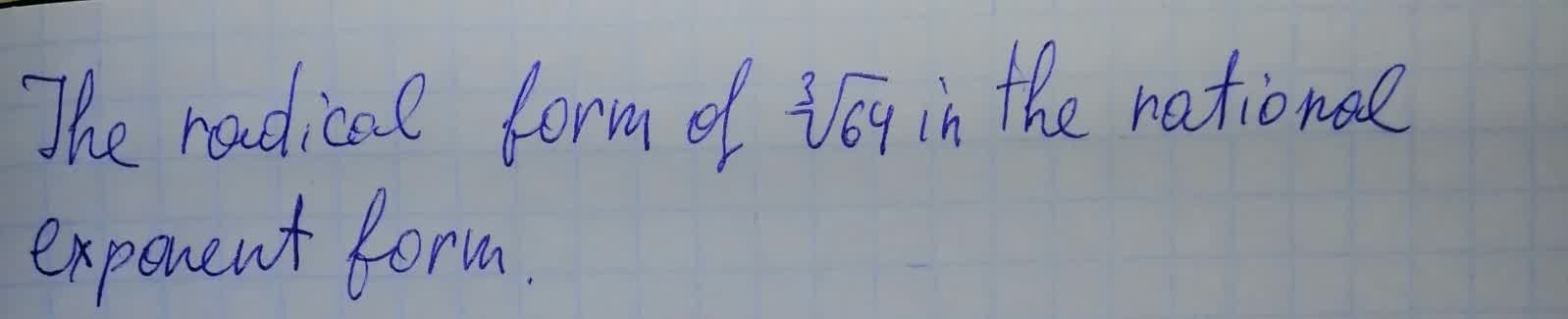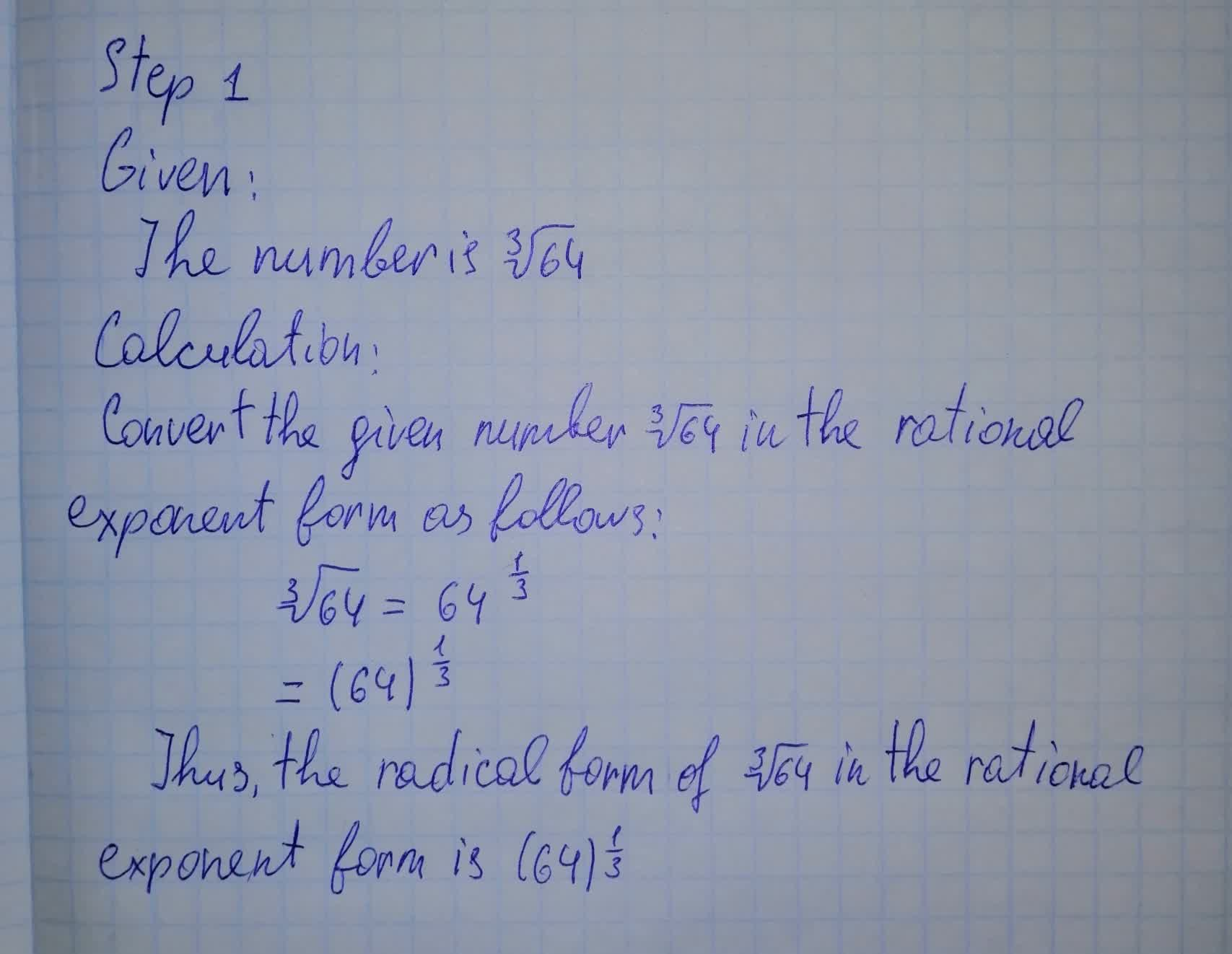# To determine: The radical form of \sqrt{64} in the rational exponent formJaya Legge 2021-08-15 Answered

To determine: The radical form of $\sqrt{64}$ in the rational exponent form.You can still ask an expert for help

• Questions are typically answered in as fast as 30 minutes

Solve your problem for the price of one coffee

• Math expert for every subject
• Pay only if we can solve itWillie###### Not exactly what you’re looking for?

$\sqrt{64}$

Rewrite 64 as ${4}^{3}$

$\sqrt{{4}^{3}}$

Pull terms out from under the radical.

4